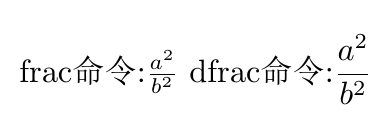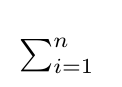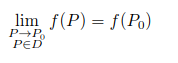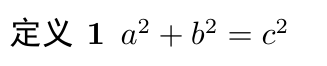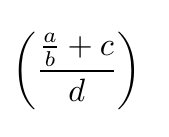# LaTeX常用命令(3)

• $…….$
• (…….)
• \begin{math}……..\end{math}

• $$……..$$
• [……….]
• \begin{displaymath}…..\end{displaymath}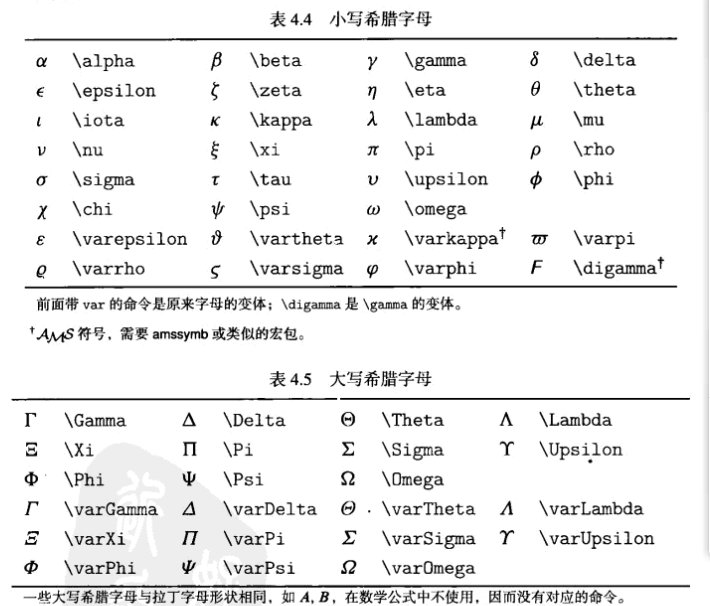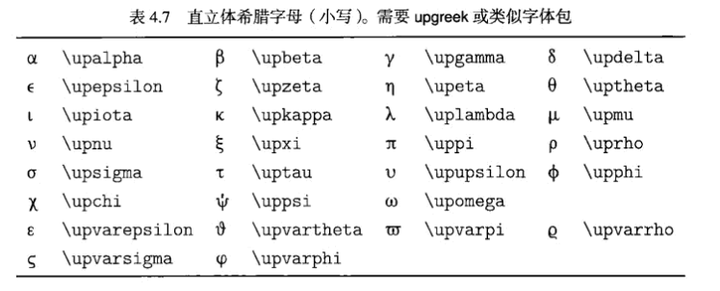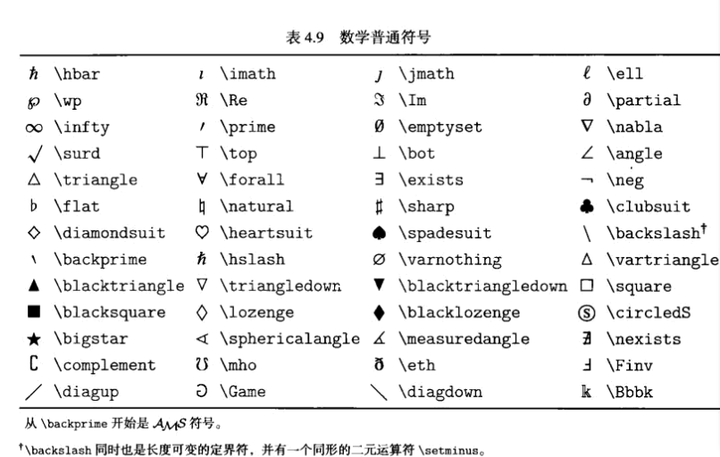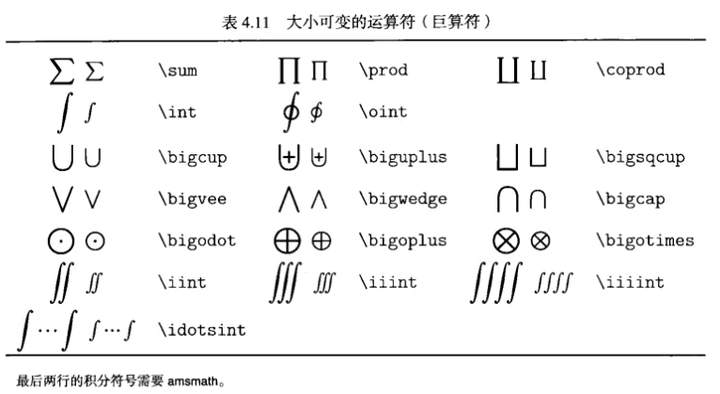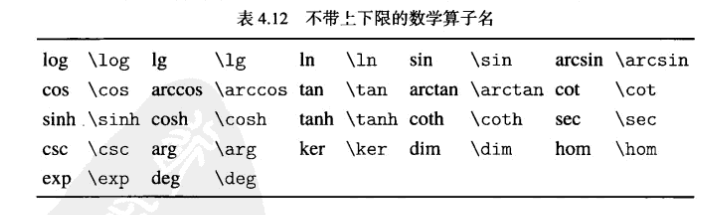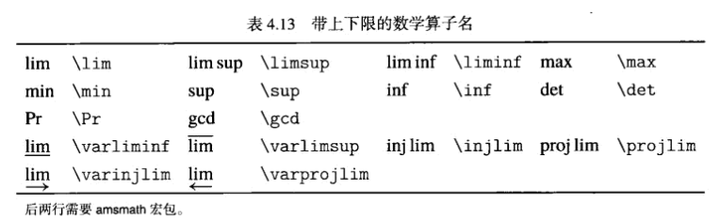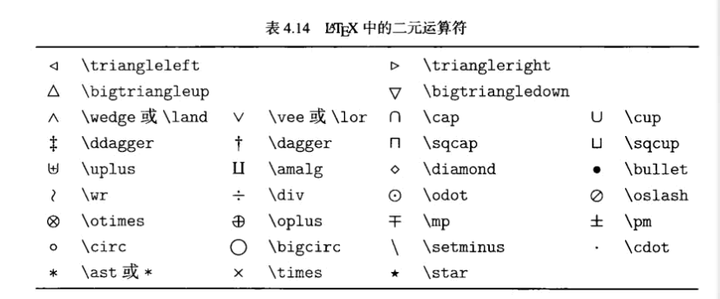\boldmath 用来加粗数学符号，如向量。需用在数学模式之外。例：\boldmath$\alpha$

\frac 与\dfrac

\frac生成的公式会在高度上压缩，\dfrac可以避免这个问题。